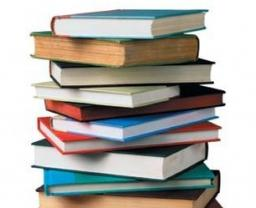# Encyclopedias 6325

The shelf contains 27 atlases, 29 dictionaries, eight textbooks, and 16 encyclopedias. What is the probability that a randomly selected book from this shelf is an encyclopedia? Give the result as a percentage.

p =  20 %

### Step-by-step explanation:Did you find an error or inaccuracy? Feel free to write us. Thank you!

Tips for related online calculators
Our percentage calculator will help you quickly calculate various typical tasks with percentages.
Would you like to compute the count of combinations?